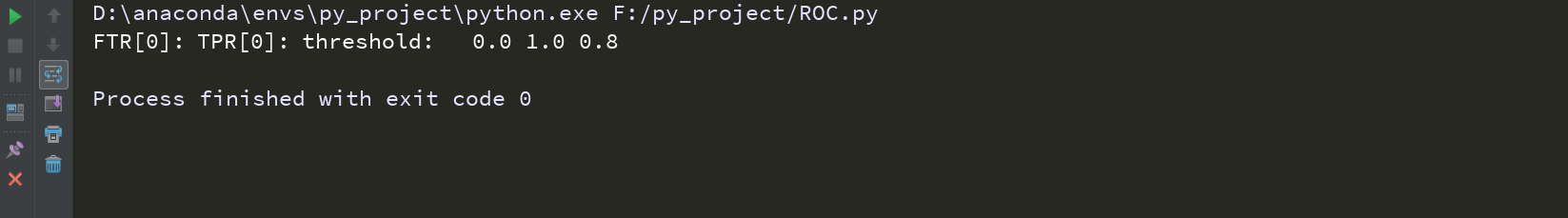# sklearn中roc_curve函数理解

### 背景介绍：

ROC受试者工作特征（ROC）曲线是另一个二分类器常用的工具。它非常类似与准确率/召回率曲

TPR：真正例率（也称召回率）TPR=TP/（TP+FN）（TP：真的被识别真的，FN：真的被识别假的）

FPR：假阳率 FPR=FP/（FP+FN）

def roc_curve(y_true, y_score, pos_label=None, sample_weight=None,drop_intermediate=True):

y_true:实际的样本标签值（这里只能用来处理二分类问题，即为{0，1}或者{true，false}，如果有多个标签，则需要使用pos_label 指定某个标签为正例，其他的为反例）

y_score:目标分数，被分类器识别成正例的分数（常使用在method="decision_function"、method="proba_predict"）

pos_label:指定某个标签为正例

from sklearn.metrics import roc_curve
import numpy as np
really_label= np.array([1, 1, 3, 2])
"""
really_label为实际的标签值，roc_curve计算的二分类的roc曲线，因此我们可以假设某一个标签为正例

"""
predicts_score=np.array([0.1, 0.4, 0.35, 0.8])
"""
predicts_score为该列上的数据被预测成改列上所对应类的概率
"""

FTR,TPR,threshold=roc_curve(really_label,predicts_score,pos_label=2)
"""
threshold 为predicts_score的逆序排列
threshold=[0.8,0.4,0.35,0.1]

predicts=[0,0,0,1]
TPR（真正率）=（1/(1+0)）=1
FNR（假阴率）=（0/（0+1））=0

TPR=0.25
FNR=0.75
threshold=0.8
"""
print("FNR:\tTPR:\tthreshold:\t",FTR,TPR,threshold)